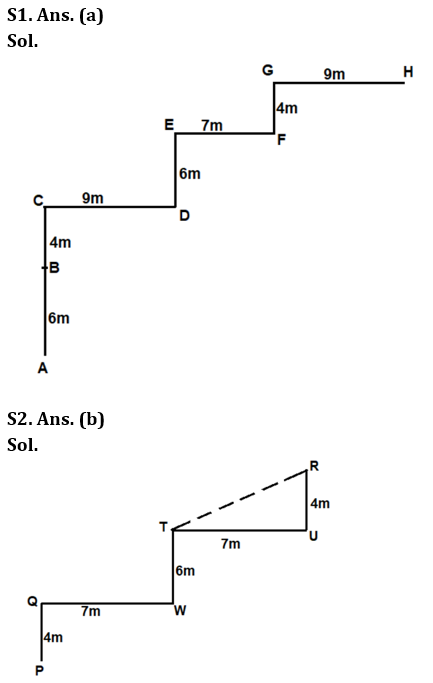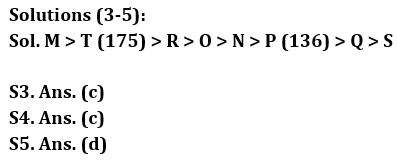Latest Banking jobs   »

# Reasoning Ability Quiz For LIC AAO Mains 2023-14th March

Directions (1-2): Answer the questions based on the information given below.
In coded language,
X&Y means Y is 6m north of X.
X\$Y means X is 4m south of Y.
X#Y means Y is 9m east of X.
X@Y means X is 7m west of Y.

Q1. In expression A&B\$C#D&E@F\$G#H, what is the direction of H with respect of A?
(a) North-east
(b) North-west
(c) East
(d) South-east
(e) None of the above

Q2. In expression P\$Q@W&T@U\$R, what is the shortest distance between T and R?
(a) √85m
(b) √65m
(c) √75m
(d) 8m
(e) None of the above

Directions (3-5): Answer the questions based on the information given below.
Eight persons P, M, N, O, Q, R, S, and T have different heights. O is % P who is % Q. R is % P but @ M. O is @ R. S is @ Q and N is @ O but % P. T is @ M but % R. Height of the one who is second tallest is 175 cm and the one who is third shortest is 136 cm.
Note – % means ‘taller than’ and @ means ‘shorter than’

Q3. If O’s height is 170m then what can be the possible height of R?
(a) 176cm
(b) 179m
(c) 172cm
(d) 177cm
(e) None of the above

Q4. How many persons are shorter than M but taller than Q?
(a) Three
(b) Four
(c) Five
(d) Six
(e) None of the above

Q5. What can be the possible height of S?
(a) 138cm
(b) 140m
(c) 142cm
(d) 124cm
(e) None of the above

Directions (6-10): Read the given arrangement of letters and numbers carefully and answer the questions based on it.

5 H K 8 2 L P 7 D W F A I Z 31 5 G Q W R X T Z 4 9 6 M N

Q6. In the given series, how many such numbers are immediately preceded by prime number?
(a) Three
(b) One
(c) Four
(d) Two
(e) None of these

Q7. In the given series, if all the alphabets which are immediately succeeded by even numbers, are removed then which element will be 15th from the left end?
(a) 5
(b) Q
(c) 3
(d) 1
(e) None of these

Q8. In the given series, how many alphabets (according to alphabetical series) are in between the sixth alphabet from the left end and eighth alphabet from the right end?
(a) 3
(b) 7
(c) 5
(d) 8
(e) None of these

Q9. What is the sum of all the even numbers which are immediately preceded by or succeeded by an alphabet in the given series?
(a) 28
(b) 30
(c) 20
(d) 34
(e) None of these

Q10. Which of the following element is 7th to the right of 16th element from the left end in the given series?
(a) 9
(b) Z
(c) X
(d) T
(e) None of these

Direction (11-15): A word and number arrangement machine when given an input line of words and numbers rearranges then following a particular rule in each step. The following is an illustration of input and rearrangement (All the numbers are two-digit numbers).

Input: 85 19 sun 52 own these ample 57 narrow 11 buy 29
Step I: 11 85 19 sun 52 own these 57 narrow buy 29 ample
Step II: 52 11 85 19 sun these 57 narrow buy 29 ample own
Step III: 19 52 11 85 sun these 57 narrow 29 ample own buy
Step IV: 29 19 52 11 85 sun these 57 ample own buy narrow
Step V: 57 29 19 52 11 85 these ample own buy narrow sun
Step VI: 85 57 29 19 52 11 ample own buy narrow sun these

Step VI is the last step of the above input. As per the rules followed in the above steps, find out in each of the following questions, the appropriate answer.

Input: 68 won appear 76 those now alone 66 52 is 92

Q11. Which word/number would be at fifth position from the right end in step III?
(a) 76
(b) Those
(c) Won
(d) Alone
(e) None of these

Q12. Which step number would be the following output?
68 76 66 92 52 those alone appear is now won
(a) IV
(b) VI
(c) V
(d) III
(e) None of these

Q13. Which of the following would be step IV?
(a) 76 66 92 52 68 won those alone appear is now
(b) 76 92 66 52 68 won those alone appear is now
(c) 76 66 92 68 52 won those alone appear is now
(d) 76 66 92 52 68 those won alone appear is now
(e) None of these

Q14. In step III, If ’92’ is related to ’68’ in a certain way and ’52’ is related to ‘won’ in the same way, which of the following would ‘those’ be related to following the same way?
(a) Now
(b) 76
(c) Appear
(d) Is
(e) Alone

Q15. Which of the following will be last step of the rearrangement?
(a) V
(b) VII
(c) VI
(d) VIII
(e) None of these

SolutionsSolutions(6-10):
S6. Ans. (b)
Sol. Given series – 5 H K 8 2 L P 7 D W F A I Z 31 5 G Q W R X T Z 4 9 6 M N
Hence, there is only one such number which is immediately preceded by prime number.

S7. Ans. (d)
Sol. Given series – 5 H K 8 2 L P 7 D W F A I Z 31 5 G Q W R X T Z 4 9 6 M N
After removing – 5 H 8 2 L P 7 D W F A I Z 31 5 G Q W R X T 4 9 6 M N
So, 15th element from the left end = 1

S8. Ans. (c)
Sol. Given series – 5 H K 8 2 L P 7 D W F A I Z 31 5 G Q W R X T Z 4 9 6 M N
Sixth alphabet from the left end = W
Eighth alphabet from the right end = Q
Hence, there are five alphabets between W and Q.

S9. Ans. (c)
Sol. Given series – 5 H K 8 2 L P 7 D W F A I Z 31 5 G Q W R X T Z 4 9 6 M N
So, required sum = 8 + 2 + 4 + 6 = 20.

S10. Ans. (d)
Sol. Given series – 5 H K 8 2 L P 7 D W F A I Z 31 5 G Q W R X T Z 4 9 6 M N
16th element from the left end = 1
So, 7th element to the right of 1 = T

Solutions (11-15):
Sol. One number and one word is arranged in each step.
In the arrangement, numbers are arranged according to the increasing order of the sum of two-digit number from left end in each step.
Words are arranged as per its first letter (followed by second letter if first letter is same) from the right end in every step. First vowel and then consonant as they appear in alphabet series.

Input: 68 won appear 76 those now alone 66 52 is 92
Step I: 52 68 won appear 76 those now 66 is 92 alone
Step II: 92 52 68 won 76 those now 66 is alone appear
Step III: 66 92 52 68 won 76 those now alone appear is
Step IV: 76 66 92 52 68 won those alone appear is now
Step V: 68 76 66 92 52 won alone appear is now those
Step VI: 68 76 66 92 52 alone appear is now those won

S11. Ans. (b)
S12. Ans. (e)
S13. Ans. (a)
S14. Ans. (e)
S15. Ans. (c)## FAQs

### When will the LIC AAO Mains exam be held?

LIC AAO Mains exam will be held on 18 March

#### Congratulations!Union Budget 2023-24: Free PDF